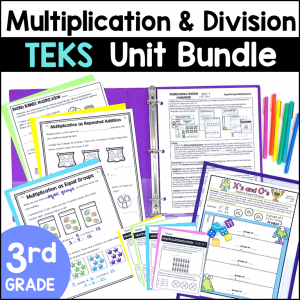Save 10% on your first order with code EXTRA10

# 3rd Grade Multiplication & Division No Prep Games

\$2.00\$29.99\$151.25
SKU: 2372425 Category:

## Description

These super fun No Prep Games are perfect to practice Multiplication and Division skills! Your students will love the fun & engaging games to practice multiplication and division concepts. Students get fun practice with arrays, jumps on a number line, relationships between multiplication and division, division word problems and much more!

What’s Included:

• 10 Games
• Color and Blackline Versions of Each Game
• Keys for most games to make self-checking math stations

Games Included:

1. X’s and O’s: Making Equal Groups Multiplication
2. Leap Frog: Multiplication on a Number Line
3. Even & Odd 4-in-a-Row: Identifying Even and Odd Numbers
4. Space Race: Area Models
5. Array Bumps: Represent Multiplication with Arrays
6. So Many Stickers: Multiplication as a Comparison Problems
7. Division 3-in-a-Row: Relating Division to Multiplication, Skip Counting & Repeated Subtraction
8. Think Multiplication 4-in-a-Row: Solving Division Problems with Related Multiplication Facts
9. Race to the Unkown: Finding the missing number in any position in multiplication or division equations
10. Division Story Bump: Solving 1-step Division Word Problems

Topics include:

• Arrays and Area Models
• Jumps on a Number Line
• Commutative Property of Multiplication
• Distributive Property of Multiplication
• Understanding the Multiplication Chart
• Relationships between Multiplication and Division
• One-step Division Word Problems
• Division of objects into Equal Groups or taking out Equal Sets
• Representing Division with Repeated Subtraction, Skip Counting, Backwards Jumps on a Number Line, and Arrays
• Even and Odd Numbers
• Describing Multiplication as a Comparison
• Finding Missing Numbers in Multiplication and Division equations

Common Core Alignment:

3.OA.A.1 Interpret products of whole numbers, e.g., interpret 5 × 7 as the total number of objects in 5 groups of 7 objects each.

3.OA.A.2 Interpret whole-number quotients of whole numbers, e.g., interpret 56 ÷ 8 as the number of objects in each share when 56 objects are partitioned equally into 8 shares, or as a number of shares when 56 objects are partitioned into equal shares of 8 objects each.

3.OA.A.3 Use multiplication and division within 100 to solve word problems in situations involving equal groups, arrays, and measurement quantities

3.OA.A.4 Determine the unknown whole number in a multiplication or division equation relating three whole numbers. For example, determine the unknown number that makes the equation true in each of the equations 8 × ? = 48, 5 = _ ÷ 3, 6 × 6 = ?

3.OA.B.6 Understand division as an unknown-factor problem. For example, find 32 ÷ 8 by finding the number that makes 32 when multiplied by 8.

3.OA.C.7 Fluently multiply and divide within 100, using strategies such as the relationship between multiplication and division

3.MD.C.7.A Find the area of a rectangle with whole-number side lengths by tiling it, and show that the area is the same as would be found by multiplying the side lengths.

TEKS Alignment:

3.4D Determine the total number of objects when equally sized groups of objects are combined or arranged in arrays up to 10 by 10.

3.4E Represent multiplication facts by using a variety of approaches such as repeated addition, equal-sized groups, arrays, area models, equal jumps on a number line, and skip counting.

3.4F Recall facts to multiply up to 10 by 10 with automaticity and recall the corresponding division facts.

3.4H Determine the number of objects in each group when a set of objects is partitioned into equal shares or a set of objects is shared equally.

3.4I Determine if a number is even or odd using divisibility rules.

3.4J Determine a quotient using the relationship between multiplication and division.

3.5C Describe a multiplication expression as a comparison such as 3 x 24 represents 3 times as much as 24.

3.5D Determine the unknown whole number in a multiplication or division equation relating three whole numbers when the unknown is either a missing factor or product.